# 题目描述（中等难度）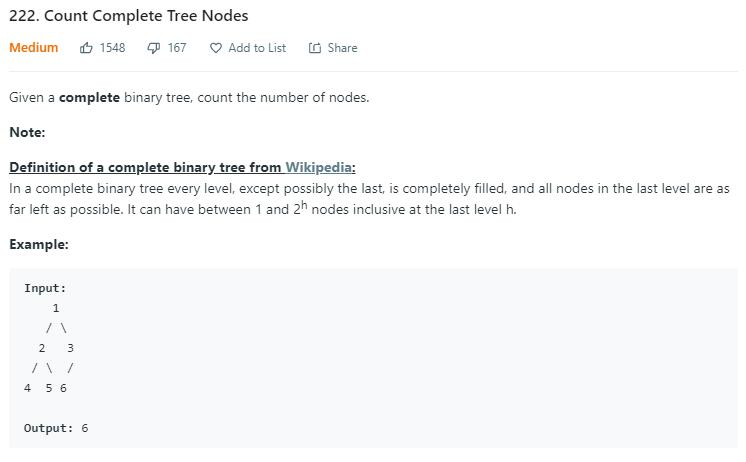# 解法之前

• full binary tree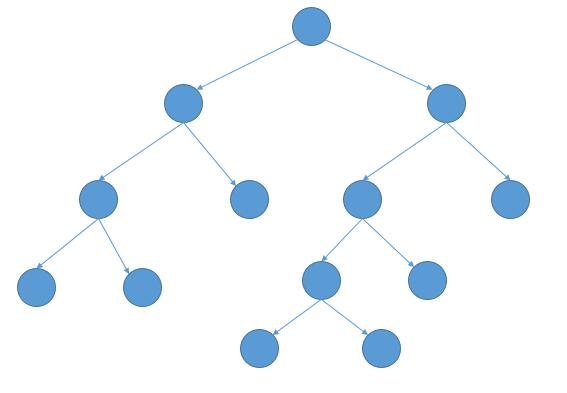下边是维基百科的定义。

A full binary tree (sometimes referred to as a proper or plane binary tree) is a tree in which every node has either 0 or 2 children. Another way of defining a full binary tree is a recursive definition. A full binary tree is either:

• A single vertex.
• A tree whose root node has two subtrees, both of which are full binary trees.

每个节点有 02 个子节点。

• perfect binary tree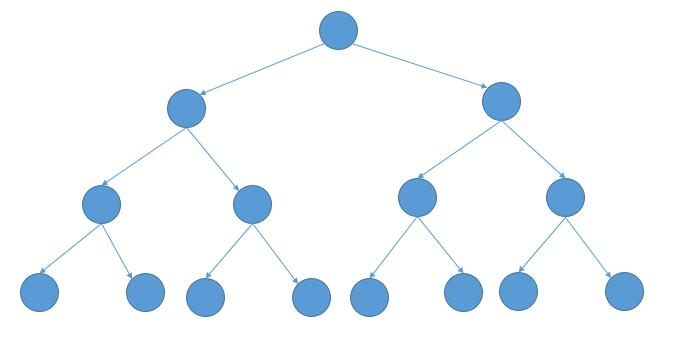下边是维基百科的定义。

A perfect binary tree is a binary tree in which all interior nodes have two children and all leaves have the same depth or same level.An example of a perfect binary tree is the (non-incestuous) ancestry chart of a person to a given depth, as each person has exactly two biological parents (one mother and one father). Provided the ancestry chart always displays the mother and the father on the same side for a given node, their sex can be seen as an analogy of left and right children, children being understood here as an algorithmic term. A perfect tree is therefore always complete but a complete tree is not necessarily perfect.

除了叶子节点外，所有节点都有两个子节点，并且所有叶子节点拥有相同的高度。

• complete binary tree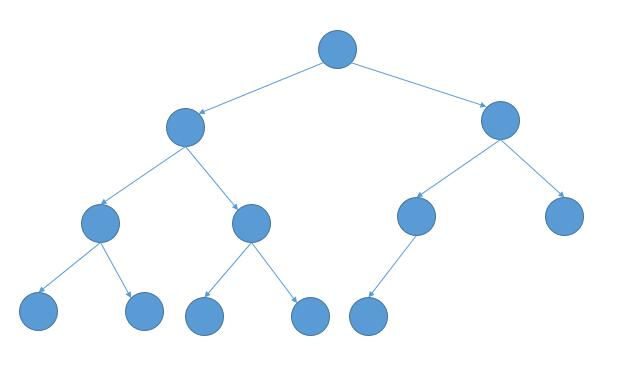下边是维基百科的定义。

In a complete binary tree every level, except possibly the last, is completely filled, and all nodes in the last level are as far left as possible. It can have between 1 and 2h nodes at the last level h. An alternative definition is a perfect tree whose rightmost leaves (perhaps all) have been removed. Some authors use the term complete to refer instead to a perfect binary tree as defined below, in which case they call this type of tree (with a possibly not filled last level) an almost complete binary tree or nearly complete binary tree. A complete binary tree can be efficiently represented using an array.

除去最后一层后就是一个 perfect binary tree，并且最后一层的节点从左到右依次排列。

11 个节点，第22 个节点，第34 个节点，...，第h$2^{h - 1}$ 个节点。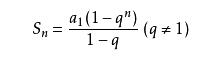# 解法一

public int countNodes(TreeNode root) {
if (root == null) {
return 0;
}
return countNodes(root.left) + countNodes(root.right) + 1;
}


public int countNodes(TreeNode root) {
if (root == null) {
return 0;
}
//因为当前树是 complete binary tree
//所以可以通过从最左边和从最右边得到的高度判断当前是否是 perfect binary tree
TreeNode left = root;
int h1 = 0;
while (left != null) {
h1++;
left = left.left;
}
TreeNode right = root;
int h2 = 0;
while (right != null) {
h2++;
right = right.right;
}
//如果是 perfect binary tree 就套用公式求解
if (h1 == h2) {
return (1 << h1) - 1;
} else {
return countNodes(root.left) + countNodes(root.right) + 1;
}
}


public int countNodes(TreeNode root) {
if (root == null) {
return 0;
}
return countNodes(root.left) + countNodes(root.right) + 1;
}


T(n) = T(n/2) + c1 lgn
= T(n/4) + c1 lgn + c2 (lgn - 1)
= ...
= T(1) + c [lgn + (lgn-1) + (lgn-2) + ... + 1]
= O(lgn*lgn)


# 解法二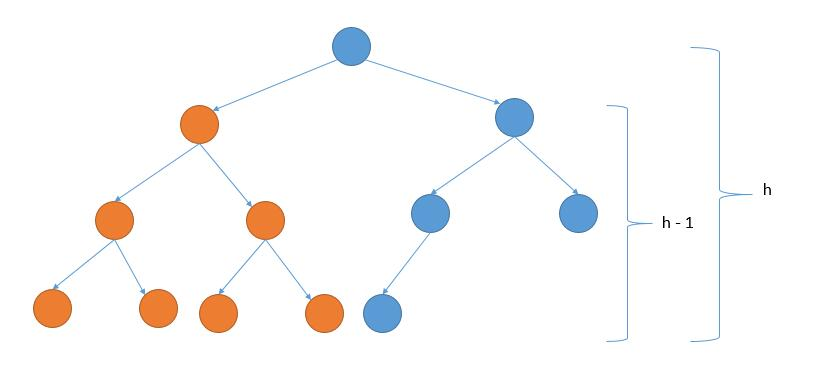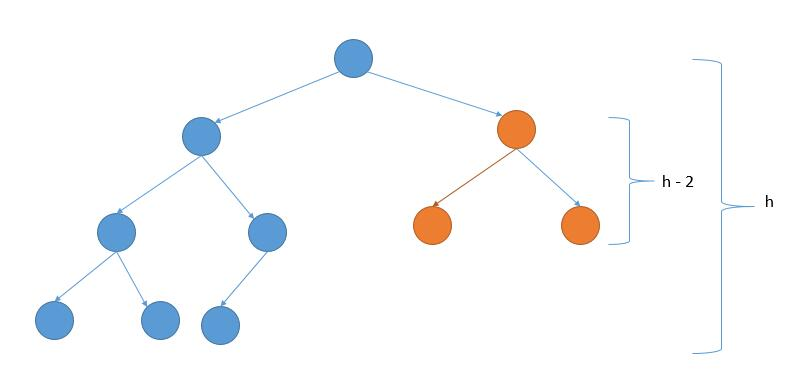private int getHeight(TreeNode root) {
if (root == null) {
return 0;
} else {
return getHeight(root.left) + 1;
}
}

public int countNodes(TreeNode root) {
if (root == null) {
return 0;
}
int height = getHeight(root);
int rightHeight = getHeight(root.right);
// 左子树是 perfect binary tree
if (rightHeight == height - 1) {
// 左子树高度和右子树高度相等
// 左子树加右子树加根节点
//return (1 << rightHeight) - 1  + countNodes(root.right) + 1;
return (1 << rightHeight) + countNodes(root.right);
// 右子树是 perfect binary tree
} else {
// 左子树加右子树加根节点
//return countNodes(root.left) + (1 << rightHeight) - 1 + 1;
return countNodes(root.left) + (1 << rightHeight);
}
}